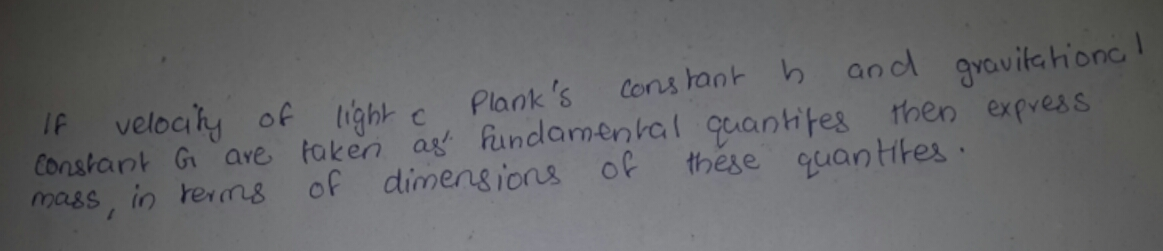# Express mass in terms of dimensions of velocity of light,Planck's constant and gravitational constant4 years ago

Here, [c]=LT1
[G]=M1L3T2
[h]=M1L2T1
Let m = cxGyhz

By substituting the dimensions of each quantity in both the sides,

M=(LT1)x(M1L3T2)y(ML2T1)z
M=[My+zLx+3y+2zTx2yz]
By equating the power of M, L, T in both the sides:

y+z=1,x+3y+2z=0,x2yz=0

By soling the above equation ,
We get, x=12,y=12,andz=12

So, [m]=c12G12h12